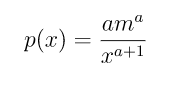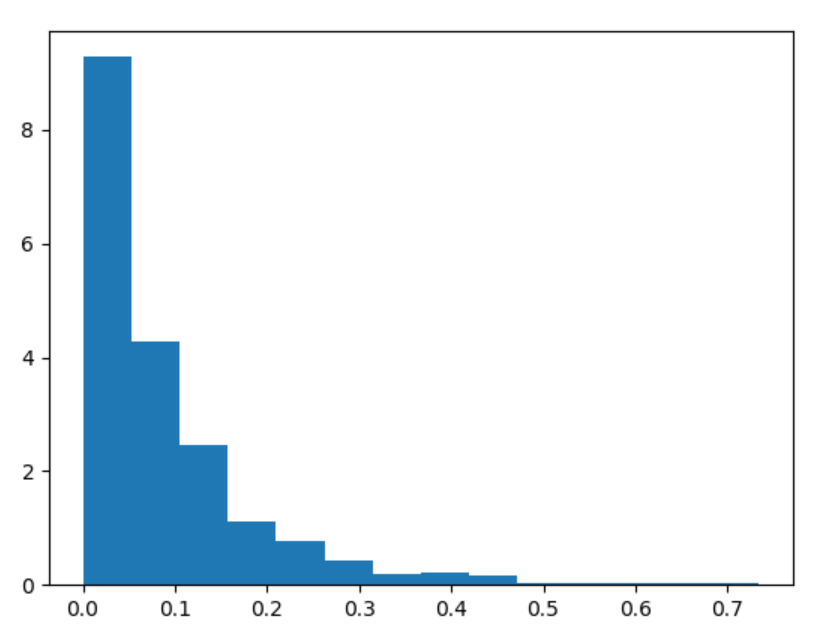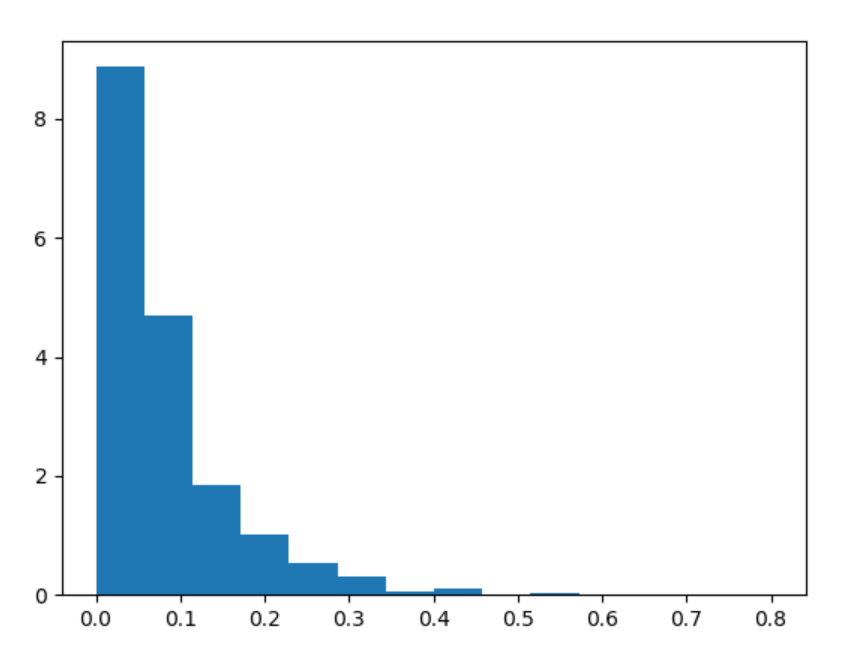# numpy.random.pareto() in Python

• Last Updated : 15 Jul, 2020

With the help of numpy.random.pareto() method, we can get the random samples from Pareto II or Lomax distribution having a specific shape and return the random samples by using this method.Lomax distribution

Syntax : numpy.random.pareto(a, size=None)

Return : Return the random samples.

Example #1 :

In this example we can see that by using numpy.random.pareto() method, we are able to get the random samples from Pareto or Lomax distribution and return the random samples by using this method.

## Python3

 `# import numpy``import` `numpy as np``import` `matplotlib.pyplot as plt`` ` `# Using numpy.random.pareto() method``gfg ``=` `np.random.pareto(``12.45``, ``1000``)`` ` `count, bins, ignored ``=` `plt.hist(gfg, ``14``, density ``=` `True``)``plt.show()`

Output :Example #2 :

## Python3

 `# import numpy``import` `numpy as np``import` `matplotlib.pyplot as plt`` ` `# Using numpy.random.pareto() method``gfg ``=` `np.random.pareto(``13.89``, ``1000``)`` ` `count, bins, ignored ``=` `plt.hist(gfg, ``14``, density ``=` `True``)``plt.show()`

Output :My Personal Notes arrow_drop_up